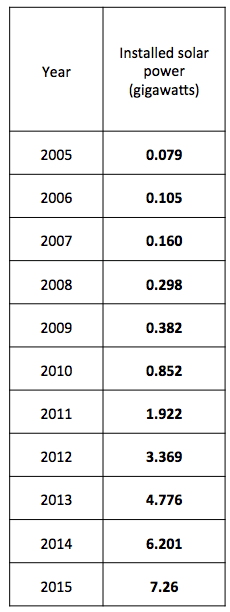# Has solar power increased in the United States?

Skills: Addition & subtraction (decimal numbers)

Related environmental issues: Energy

The circle below shows how much new solar power was installed the United States each year between 2005 and 2015.1. Has the number increased or decreased as time goes by?
2. What is the difference between the numbers of the years 2008 and 2009?
3. Is the amount of solar panels installed in 2011 more than those installed in 2009 and 2010 combined?
4. What is the difference between the largest number and the smallest number?
5. Which two numbers make the largest value when added

Sources: REN 21, 2016

__________________________________________________________